GFG App
Open AppBrowser
Continue

# Class 10 RD Sharma Solutions- Chapter 10 Circles – Exercise 10.2 | Set 2

### Question 11. In the figure, PQ is tangent at a point R of the circle with centre O. If ∠TRQ = 30°, find m ∠PRS

Solution: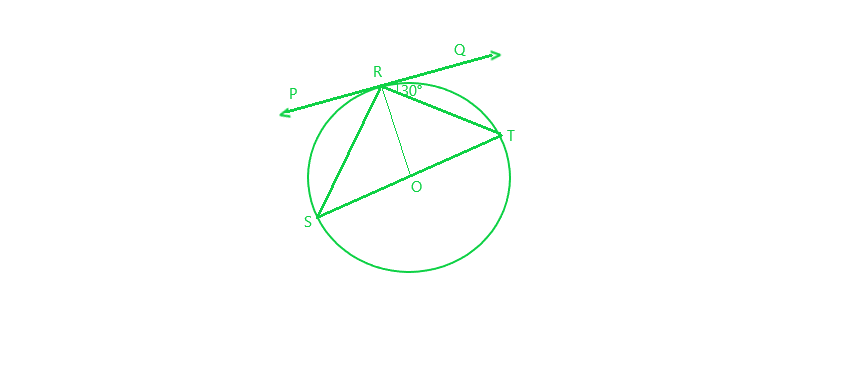∠TRQ = 30° and PQ is tangent at point R

To Find: ∠PRS

Now ∠SRT = 90°     (angle in a semicircle)

Also, ∠TRQ + ∠SRT + ∠PRS = 180°     (Angles of a line)

=> 30° + 90° + ∠PRS = 180°

=> ∠PRS = 180° – 120°

=> ∠PRS = 60°

### Question 12. If PA and PB are tangents from an outside point P, such that PA = 10 cm and ∠APB = 60°. Find the length of chord AB.

Solution: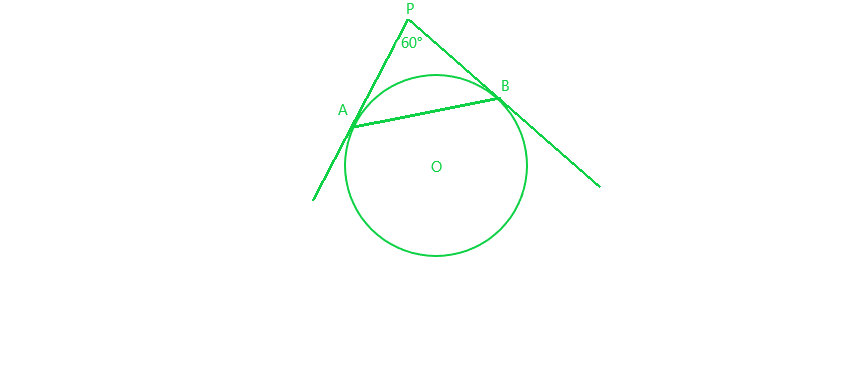PA = 10 cm and ∠APB = 60°

We also know that

PA = PB = 10 cm     (Tangents drawn from a point outside the circle are equal)

=> ∠PAB = ∠PBA

Now, In ∆APB, we have:

∠APB + ∠PAB + ∠PBA = 180°     (Angles of a triangle)

=> 60° + ∠PAB + ∠PAB = 180°

=> 2 ∠PAB = 180° – 60° = 120°

=> ∠PAB = 60°

∠PBA = ∠PAB = 60°

Hence. PA = PB = AB = 10 cm

Thus, length of chord AB = 10 cm

### Question 13. In a right triangle ABC in which ∠B = 90°, a circle is drawn with AB as diameter intersecting the hypotenuse AC at P. Prove that the tangent to the circle at P bisects BC.

Solution: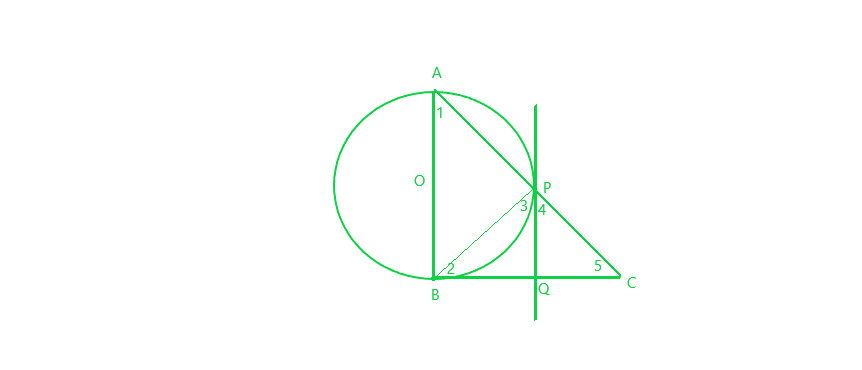Given: Let O be the centre of the circle with diameter AB.

Tangents to the circle at P meets BC at Q.

To Prove: PQ bisects BC, i.e., BQ = QC

Proof:

∠ABC = 90°

In ∆ABC, ∠1 + ∠5 = 90°     [angle sum property, ∠ABC = 90°]

∠3 = ∠1     [angle between tangent and the chord equals angle made by the chord in alternate segment]

=> ∠3 + ∠5 = 90°     (1)

And, ∠APB = 90°     [angle in semi-circle]

=> ∠3 + ∠4 = 90°     (2)     [∠APB + ∠BPC = 180°, linear pair]

From Equation (1) and (2), we get

∠3 + ∠5 = ∠3 + ∠4

∠5 = ∠4

=> PQ = QC     (3)     [sides opposite to equal angles are equal]

Also, QP = QB     (4)     [tangents drawn from an internal point to a circle are equal]

From equation (3) and (4), we get:

=> QB = QC

Thus, PQ bisects BC is proved

### Question 14. From an external point P, tangents PA and PB are drawn to a circle with centre O. If CD is the tangent to the circle at a point E and PA = 14 cm, find the perimeter of ∆PCD.

Solution: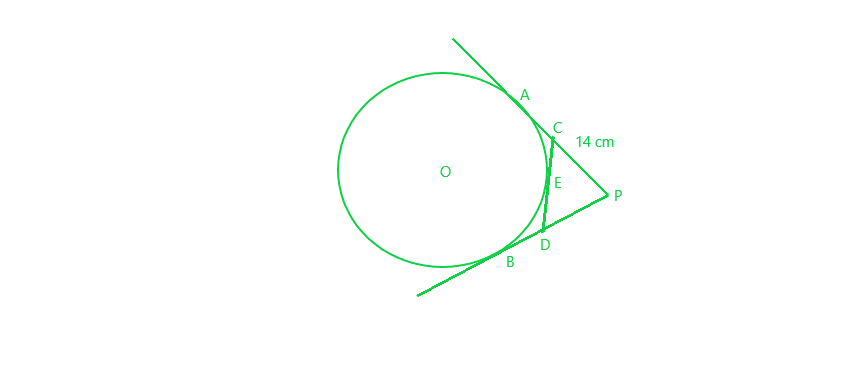PA = 14 cm

PA = PB = 14 cm     (PA and PB are the tangents to the circle from P)

Also,

CA = CE     (1)     (CA and CE are the tangents from C)

DB = DE     (2)     (DB and DE are the tangents from D)

So, perimeter of ∆PCD:

= PC + PD + CD

=> PC + PD + CE + DE

=> PC + CE + PD + DE

=> PC + CA + PD = DB     (From (1) and (2))

=> PA + PB

=> 14 cm + 14 cm

= 28 cm

### Question 15. In the figure, ABC is a right triangle right-angled at B such that BC = 6 cm and AB = 8 cm. Find the radius of its incircle.

Solution: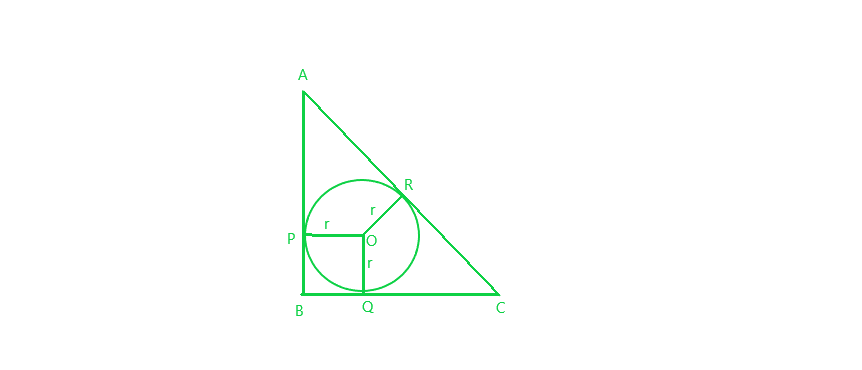∠B = 90°, BC = 6 cm, AB = 8 cm and r be the radius of incircle with centre O

Applying Pythagoras Theorem in right-angled ∆ABC:

AC² = AB² + BC²

=> AC²  = (8)² + (6)² = 64 + 36 = 100

=> AC = 10 cm

=> AR + CR = 10 cm     (1)

Now, AP = AR     (AP and AR are the tangents to the circle from A)

Similarly, CR = CQ and BQ = BP

OP and OQ are radii of the circle = r     (2)

OP ⊥ AB and OQ ⊥ BC     (3)     (angle between the radius to the point of contact of tangent is 90°)

and ∠B = 90°     (4)

From equation (2), (3) and (4):

BPOQ is a square

=> BP = BQ = r

=> AR = AP = AB – BD = 8 – r     (5)

and CR = CQ = BC – BQ = 6 – r     (6)

From equation (1), (5) and (6), we get:

AR + CR = 10

=> 8 – r + 6 – r = 10     (from (5) and (6))

=> 14 – 2r = 10

=> 2r = 14 – 10 = 4

=> r = 2

Radius of the incircle = 2 cm

### Question 16. Prove that the tangent drawn at the mid-point of an arc of a circle is parallel to the chord joining the endpoints of the arc.

Solution: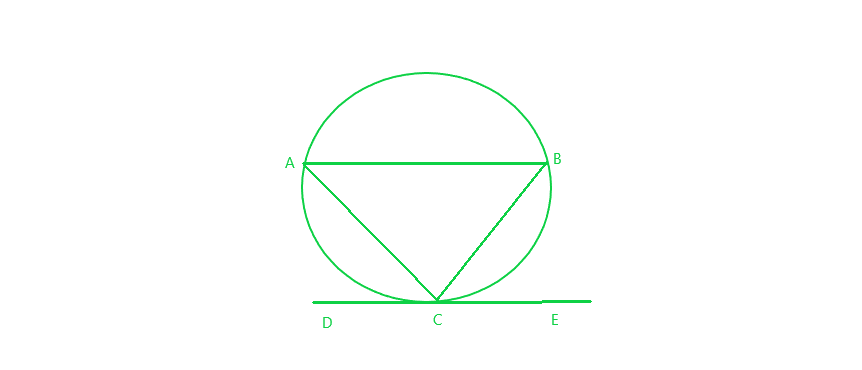Given: Let mid-point of arc ACB be C and, DCE be the tangent to it

To prove: AB || DCE

Proof:

Arc AC = Arc BC

=> Chord AC = Chord BC

Now, In ∆ABC,

AC = BC

=> ∠CAB = ∠CBA      (1)     (equal sides corresponding to the equal angle)

Since, DCE is a tangent line.

∠ACD = ∠CBA     (angle in alternate segment are equal)

=> ∠ACD = ∠CAB     (from Eq. (1))

=> ∠ACD and ∠CAB are alternate angles

Which is only possible only when AB || CDE

Hence, the tangent drawn at the mid-point of an arc of a circle is parallel to the chord joining the end points of the arc.

### Question 17. From a point P, two tangents PA and PB are drawn to a circle with centre O. If OP = diameter of the circle show that ∆APB is equilateral.

Solution:Given :  Two tangents PA and PB are drawn to the circle and OP is diameter

To prove: ∆APB is equilateral

Proof:  OP = 2r     (let r be the radius of the circle)

=> OQ + QP = 2r

=> OQ = QP = r     (OQ is the radius)

Now in right ∆OAP,

OP is the hypotenuse and Q is the mid point of it

=> OA = AQ = OQ     (mid-point of hypotenuse of a right triangle is equidistant from its vertices)

Thus, ∆OAQ is equilateral triangle

=> ∠AOQ = 60°

Also, ∠APO = 90° – 60° = 30°     (Sum of all the angles of triangle is 180° )

=> ∠APB = 2 ∠APO = 2 x 30° = 60°     (1)

We also know that, PA = PB (Tangents from P to the circle)

=> ∠PAB = ∠PBA      (2)

Now, in ∆APB:

∠PBA + PAB + ∠APB = 180°     (Sum of all angles)

=> 2∠PBA = 120°     (from (1) and (2))

=> ∠PAB = ∠PBA = 60°

Hence, ∆APB is an equilateral triangle.

### Question 18. Two tangents segments PA and PB are drawn to a circle with centre O such that ∠APB = 120°. Prove that OP = 2 AP.

Solution:Given : Two tangents to the circle from a point P and ∠APB = 120°

To prove : OP = 2 AP

Proof : In right ∆OAP,

∠OPA = (1/2)∠APB = 60°

=> ∠AOP = 90° – 60° = 30°

Let, Q be mid point of hypotenuse OP of ∆OAP

=> QO = QA = QP

=> ∠OAQ = ∠AOQ = 30°

=> ∠PAQ = 90° – 30° = 60°

So, ∆AQP is an equilateral triangle

=> QA = QP = AP     (1)

Also, Q is mid point of OP

=> OP = 2 QP = 2 AP     (from (1))

Hence, proved.

### Question 19. If ∆ABC is isosceles with AB = AC and C (0, r) is the incircle of the ∆ABC touching BC at L. Prove that L bisects BC.

Solution: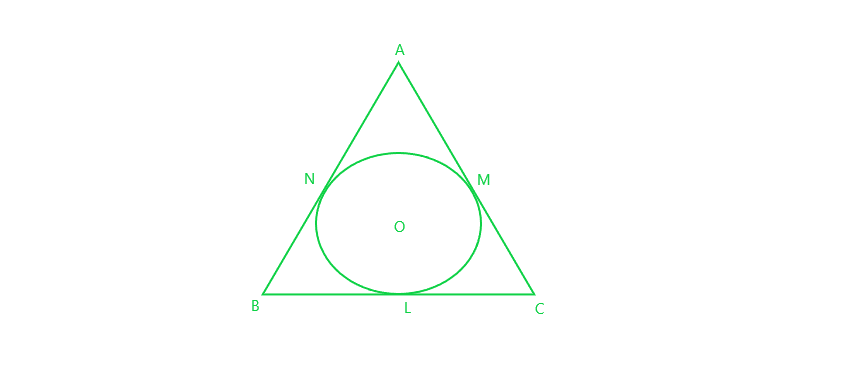Given: ∆ABC isosceles with AB = AC and incircle with centre O and radius r touches the side BC of ∆ABC at L.

To prove : L is mid point of BC.

Proof : AM and AN are the tangents to the circle from A

=> AP = AQ

But AB = AC     (given)

=> AB – AQ = AC – AP

=> BQ = CP     (1)

Now BL and BQ are the tangents from B

=> BL = BQ     (2)

Similarly, CL and CP are tangents

=> CL = CP     (3)

Also, BQ = CP     (from (1))

=> BL = CL     (from (2) and (3))

Hence, proved that L is mid point of BC.

My Personal Notes arrow_drop_up